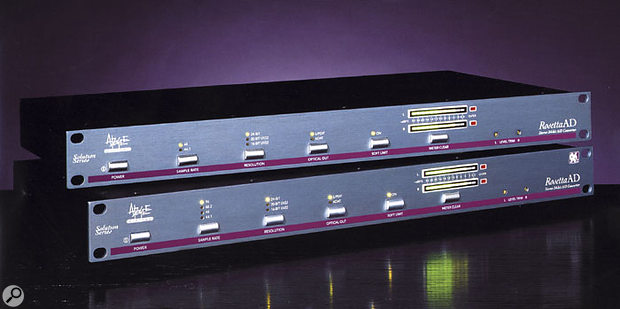# Q. How do A-D converters work?

By Hugh RobjohnsThough gearheads rarely lust after them in the way they do with other types of equipment, A-D converters, like these Apogee Rosettas, are arguably the most important tool you possess when working in the digital domain.

How does a converter actually make an analogue signal into a digitally represented signal? What are a converter's components that enable such A-D conversion?

SOS Forum Post

Technical Editor Hugh Robjohns replies: There are three steps: filtering, sampling and quantising.

First, the audio bandwidth of the analogue signal has to be defined using a low-pass filter called an anti-alias filter. This is to make sure that the signal contains no frequencies higher than slightly less than half the sampling rate. So, in the case of a 44.1kHz sampled system like CDs, the audio bandwidth would be restricted to about 21kHz.

Next, the signal is sampled at the appropriate sampling rate. In other words, the instantaneous voltage of the analogue signal is measured at regular time intervals. This stage is essentially turning the continuously varying audio waveform into a series of discrete snapshots, in much the same way that a film camera stores continuous live action as a series of still frames.

These individual audio snapshot voltages are then 'quantised.' This means measuring the fixed analogue voltage which represents the amplitude of the waveform at the moment the sample was taken, and describing its value with a binary number which can be handled by the rest of the digital system. The output from the quantiser is the digital signal that is then recorded, transmitted or used in whatever way is intended.

A two-bit system can count four discrete levels or voltages, which is obviously pretty crude. A 16-bit system can count 65,536 different levels and a 24-bit system can count over 16 million levels. Obviously, if the maximum size of the original analogue waveform remains the same, then the greater the number of bits used in the quantiser, the smaller the voltage change that can be represented. This translates to a lower noise floor (or greater dynamic range). So a two-bit system has a dynamic range of about 12dB, while a 16-bit system has a dynamic range of about 96dB and a 24-bit system theoretically reaches about 140dB.

Published November 2003